# .css-4zleql{display:block;}Rishit Dagli's Blog# Build K-Means from scratch in Python

Subscribe to my newsletter and never miss my upcoming articles

## Build K-Means from scratch in Python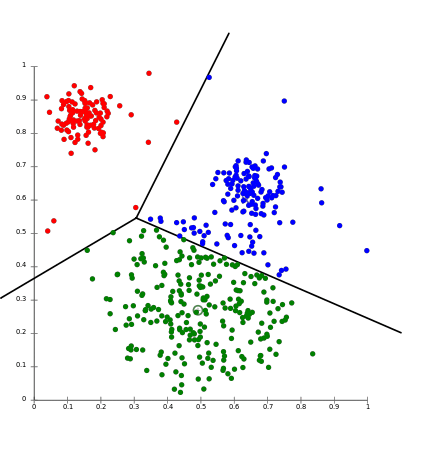## Introduction to K-means Clustering

K-means clustering is a type of unsupervised learning, which is used when you have unlabeled data (i.e., data without defined categories or groups). The goal of this algorithm is to find groups in the data, with the number of groups represented by the variable K. The algorithm works iteratively to assign each data point to one of K groups based on the features that are provided. Data points are clustered based on feature similarity. The results of the K-means clustering algorithm are:

• The centroids of the K clusters, which can be used to label new data

• Labels for the training data (each data point is assigned to a single cluster)

Rather than defining groups before looking at the data, clustering allows you to find and analyze the groups that have formed organically. The “Choosing K” section below describes how the number of groups can be determined.

This story covers:

• Why use K-means

• The steps involved in running the algorithm

• An Python example with toy data set from scratch

## Why use K-Means ?

The algorithm can be used to confirm business assumptions about what types of groups exist or to identify unknown groups in complex data sets. Once the algorithm has been run and the groups are defined, any new data can be easily assigned to the correct group.

This is a versatile algorithm that can be used for any type of grouping. Some examples of use cases are:

Behavioral segmentation:

• Segment by purchase history

• Segment by activities on application, website, or platform

• Define personas based on interests

• Create profiles based on activity monitoring

Inventory categorization:

• Group inventory by sales activity

• Group inventory by manufacturing metrics

Sorting sensor measurements:

• Detect activity types in motion sensors

• Group images

• Separate audio

• Identify groups in health monitoring

Detecting bots or anomalies:

• Separate valid activity groups from bots

• Group valid activity to clean up outlier detection

In addition, monitoring if a tracked data point switches between groups over time can be used to detect meaningful changes in the data.

## The Algorithm

K-Means is actually one of the simplest unsupervised clustering algorithm. Assume we have input data points x1,x2,x3,…,xn and value of K(the number of clusters needed). We follow the below procedure:

1. Pick K points as the initial centroids from the data set, either randomly or the first K.

2. Find the Euclidean distance of each point in the data set with the identified K points — cluster centroids.

3. Assign each data point to the closest centroid using the distance found in the previous step.

4. Find the new centroid by taking the average of the points in each cluster group.

5. Repeat 2 to 4 for a fixed number of iteration or till the centroids don’t change.

Here Let point a=(a₁,a₂) ; b=(b₁,b₂) and D be the Euclidean distance between the points then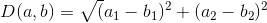Mathematically speaking, if each cluster centroid is denoted by cᵢ, then each data point x is assigned to a cluster based on

arg (min (cᵢ ϵ c) D(cᵢ,x)²)

For finding the new centroid from clustered group of points —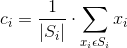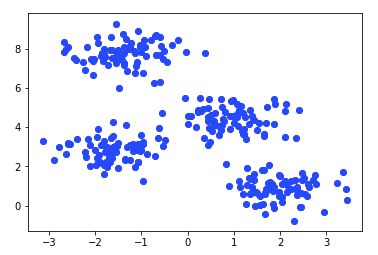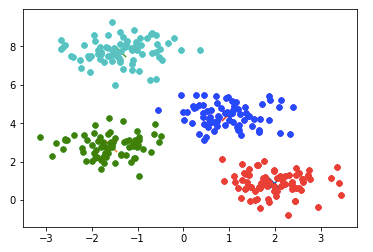## Choosing the right K

So it’s really problematic if you choose the wrong K, so this is what I am talking about.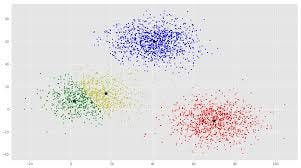So this was a clustering problem for 3 cluster, K = 3 but if I choose K = 4, I will get something like this and this will definitely not give me the kind of accuracy of predictions, I want my model too. Again if I choose K = 2, I get something like this —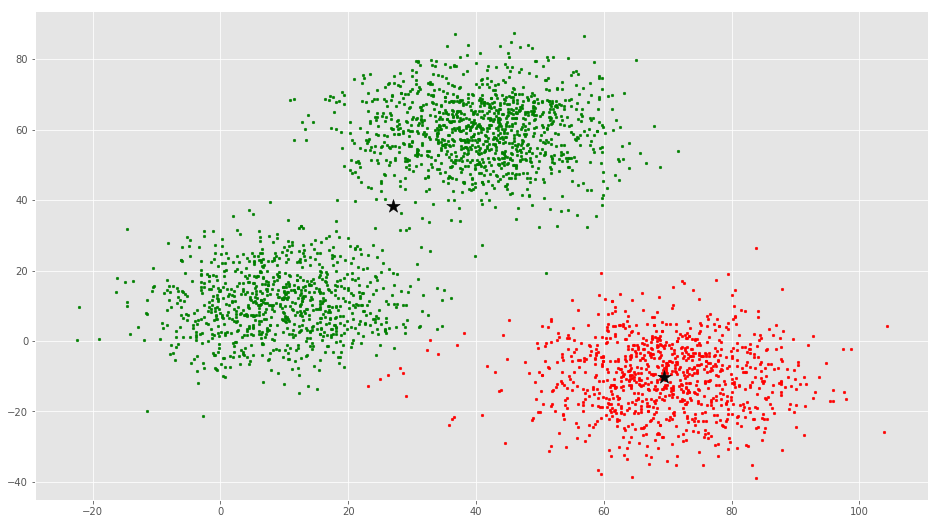Now this isn’t a good prediction boundary too. You or me can figure out the ideal K easily in this data as it is 2 dimensional, we could figure out the K for 3 dimensional data too, but lets say you have 900 features and you reduce them to around a 100 features, you cannot visually spot out the ideal K (these problems usually occur in gene data). So, you want a proper way for figuring or I would say guessing an approximate K. To do this we have may algorithms like cross validation, bootstrap, AUC, BIC and many more. But we will discuss one of the most simple and easy to comprehend algorithm called “elbow point”.

The basic idea behind this algorithm is that it plots the various values of cost with changing k. As the value of K increases, there will be fewer elements in the cluster. So average distortion will decrease. The lesser number of elements means closer to the centroid. So, the point where this distortion declines the most is the elbow point and that will be our optimal K.

For the same data shown above this will be the cost(SSE — sum of squared errors) vs K graph created —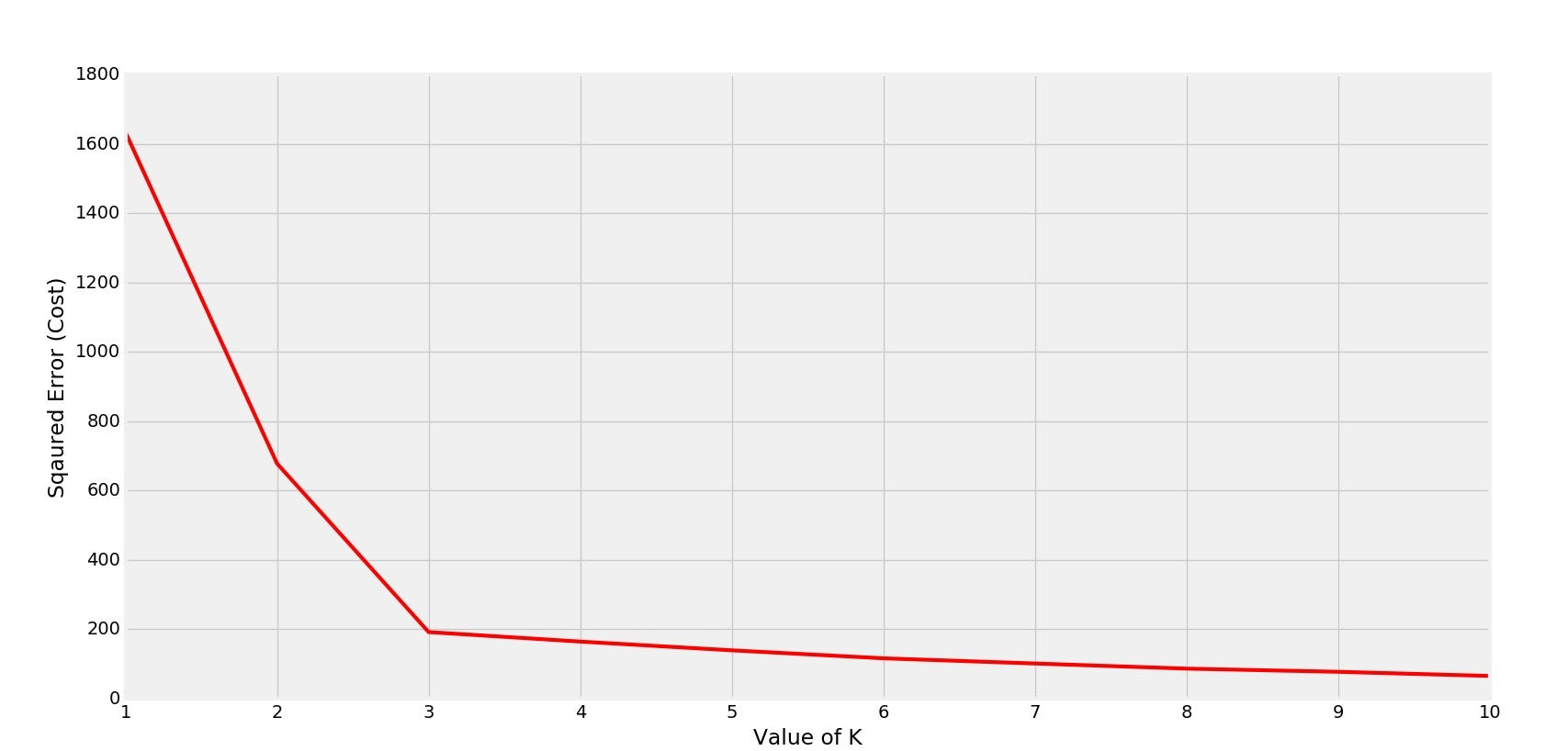So, our ideal K is 3. Let us take another example where ideal K is 4. This is the data-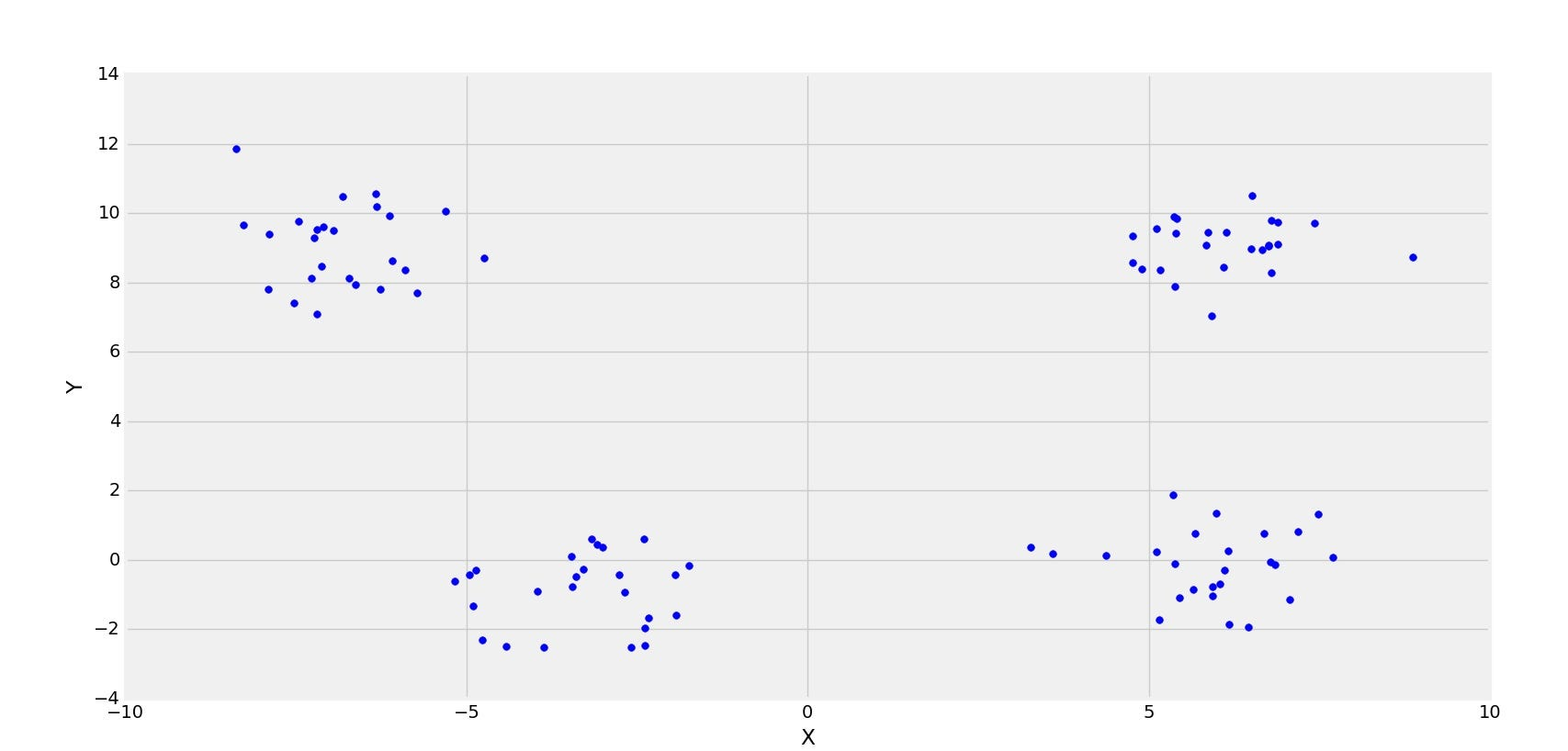And, this is the cost vs K graph created-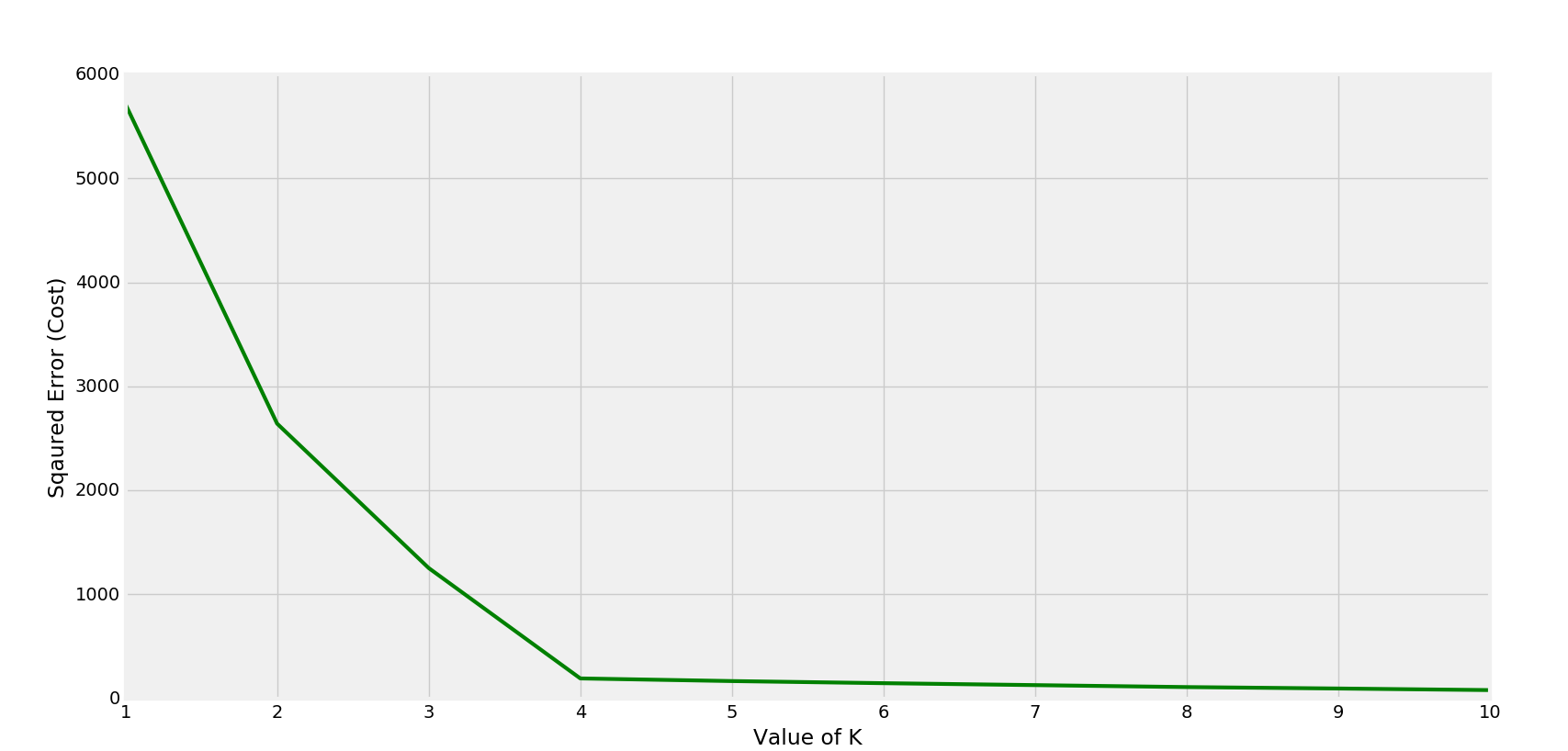So, we easily infer that K is 4.

## Python Implementation

Here we will use the libraries, Matplotlib to create visualizations and Numpy to perform calculation.

So first we will just plot our data —

``````import matplotlib.pyplot as plt
import numpy as np

from matplotlib import style
style.use('ggplot')

X = np.array([[1, 2],
[1.5, 1.8],
[5, 8 ],
[8, 8],
[1, 0.6],
[9,11]])

plt.scatter(X[:,0], X[:,1], s=150)
plt.show()
``````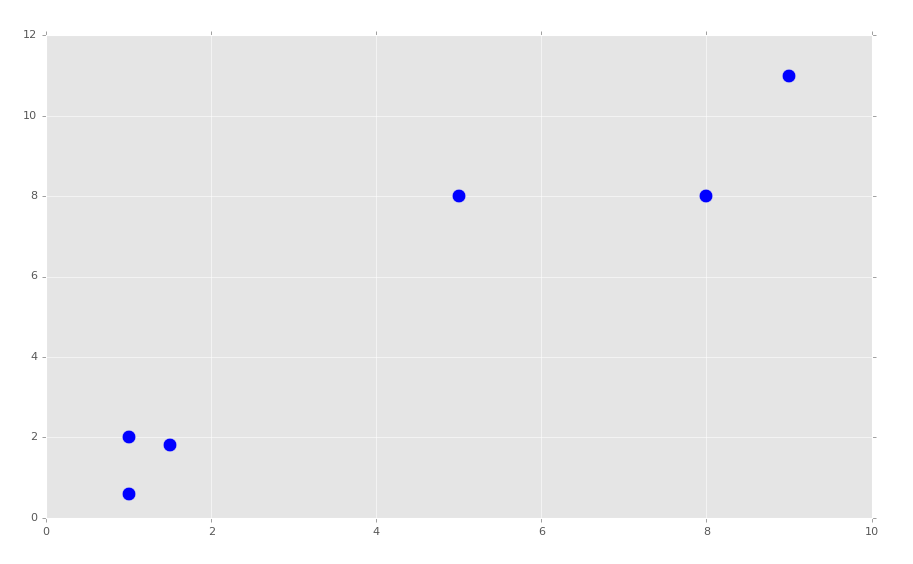To make our work organized we will build a class and proceed as in the steps above and also define a tolerance or threshold value for the SSE’s.

``````class K_Means:
def __init__(self, k=2, tol=0.001, max_iter=300):
self.k = k
self.tol = tol
self.max_iter = max_iter

def fit(self,data):

self.centroids = {}

for i in range(self.k):
self.centroids[i] = data[i]

for i in range(self.max_iter):
self.classifications = {}

for i in range(self.k):
self.classifications[i] = []

for featureset in data:
distances = [np.linalg.norm(featureset-self.centroids[centroid]) for centroid in self.centroids]
classification = distances.index(min(distances))
self.classifications[classification].append(featureset)

prev_centroids = dict(self.centroids)

for classification in self.classifications:
self.centroids[classification] = np.average(self.classifications[classification],axis=0)

optimized = True

for c in self.centroids:
original_centroid = prev_centroids[c]
current_centroid = self.centroids[c]
if np.sum((current_centroid-original_centroid)/original_centroid*100.0) > self.tol:
print(np.sum((current_centroid-original_centroid)/original_centroid*100.0))
optimized = False

if optimized:
break

def predict(self,data):
distances = [np.linalg.norm(data-self.centroids[centroid]) for centroid in self.centroids]
classification = distances.index(min(distances))
return classification
``````

Now we can do something like:

``````model = K_Means()
model.fit(X)

for centroid in model.centroids:
plt.scatter(model.centroids[centroid], model.centroids[centroid],
marker="o", color="k", s=150, linewidths=5)

for classification in model.classifications:
color = colors[classification]
for featureset in model.classifications[classification]:
plt.scatter(featureset, featureset, marker="x", color=color, s=150, linewidths=5)

plt.show()
``````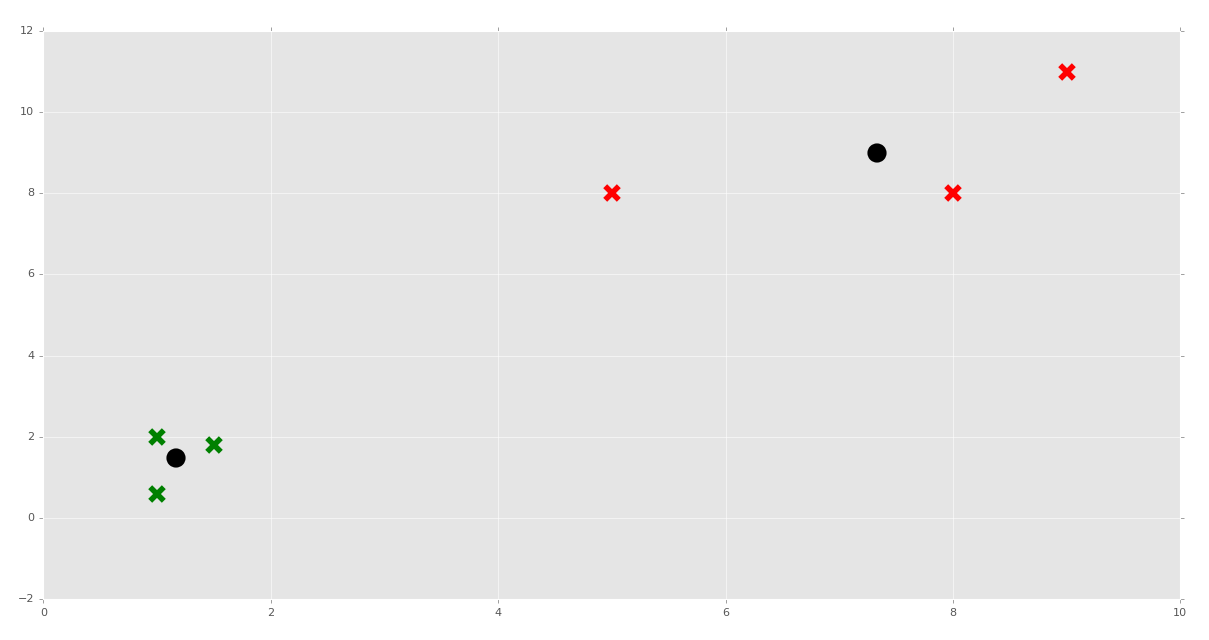Next, you can also use the predict function. So that was about K-Means and we are done with the code 😀